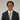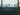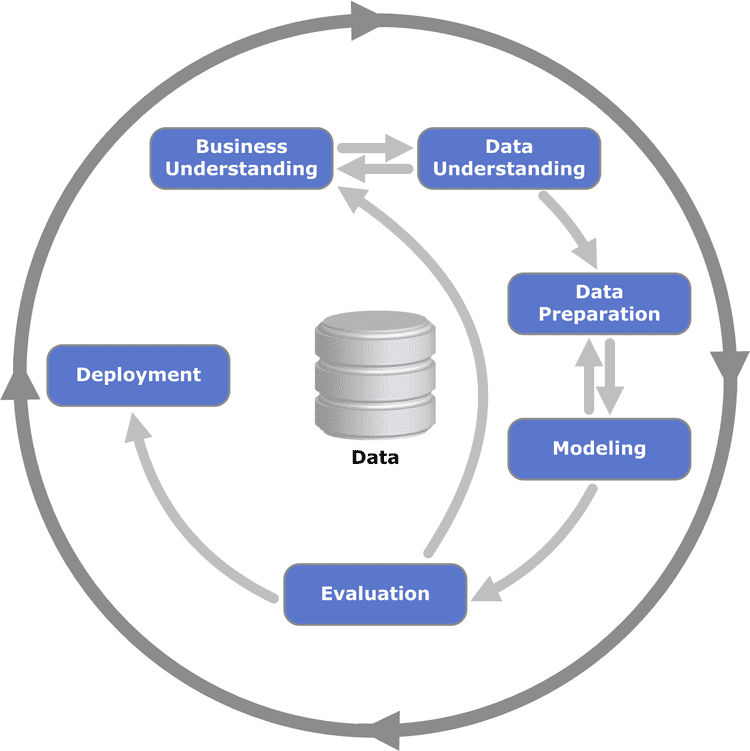Navigate back to the homepage

Using d6tflow in Python to Simplify Data Science Pipelines and Develop FasterJack Moody
November 9th, 2020 · 3 min readData Science: A Quick Overview

At a basic level, the data science process looks something like this:• Source raw data to help solve problem/investigate hypothesis
• Clean data into a usable format
• Explore data to understand what you are working with
• Analyze and model data to try to find relationships between variables

Python has become a popular language for data science as a result of its simple syntax, object-oriented nature, and amazing open-source community. However, Python on its own does not lend itself well to data science. That is where the power of open-source projects such as pandas, numpy, sklearn, and countless other projects come into play.

Predicting Boston House Prices: The Hard Way

A basic data science workflow might look something like this:

1import pandas as pd
3from sklearn.model_selection import train_test_split
4from sklearn.linear_model import LinearRegression
5
8X = pd.DataFrame(boston.data, columns=boston.feature_names)
9y = pd.DataFrame(boston.target, columns=['Price'])
10
11# Break into training and testing data
12X_train, X_test, y_train, y_test = train_test_split(X, y)
13
14# Model data
15reg = LinearRegression()
16reg.fit(X_train, y_train)
17
18# Test model
19reg.score(X_test, y_test)

The code above is great for short data science pipelines. However, you probably want to do more than just try a linear regression model. There are lots of different model types that could be fitted to this data. How can we change the code above to run a SVM regression or a decision tree regression?

Well, that isn't too bad. We just add the following few lines:

1from sklearn.tree import DecisionTreeRegressor
2from sklearn.svm import SVR
3
4# Model data
5reg_dt = DecisionTreeRegressor()
6reg_svr = SVR()
7
8reg_dt.fit(X_train, y_train)
9reg_svr.fit(X_train, y_train)
10
11# Test models
12reg_dt.score(X_test, y_test)
13reg_svr.score(X_test, y_test)

The code above is quickly starting to look repetitive. Let's make functions to reduce our code duplication.

1def train_and_score(model, X_train, y_train, X_test, y_test):
2 model.fit(X_train, y_train)
3 return model.score(X_test, y_test)
4
5train_and_score(LinearRegression(), X_train, y_train, X_test, y_test)
6train_and_score(DecisionTreeRegressor(), X_train, y_train, X_test, y_test)
7train_and_score(SVR(), X_train, y_train, X_test, y_test)